# OECD Meat Consumption Data Analysis 7 - Time Series Regression using R dynlm() function.Photo by Ashutosh Saraswat on Unsplash

This post is following of aabove post.
In this post, I will do some time-series regression with R.

First, I made JPN only dataframe.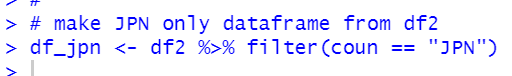Let's see df_jpn.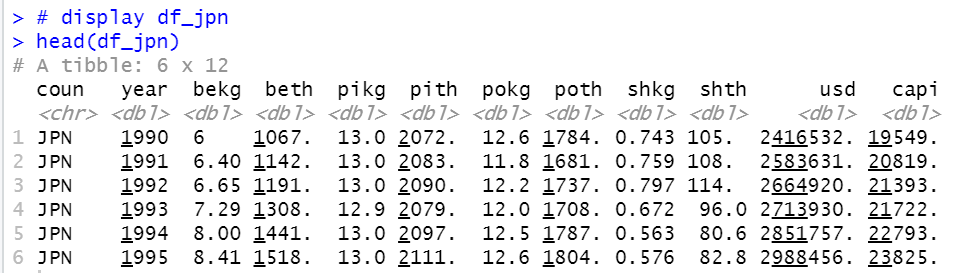Then, I make ts object form df_jpn.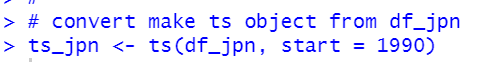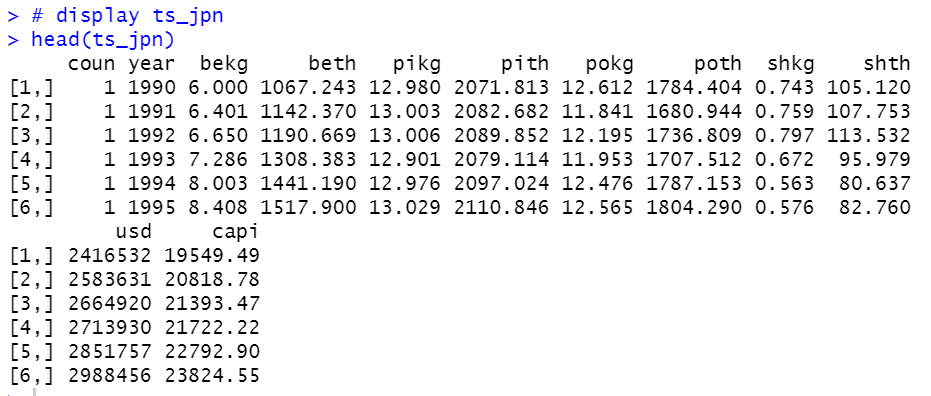Let's see pokg and capi.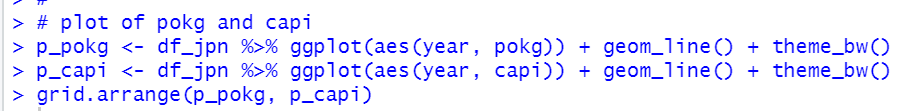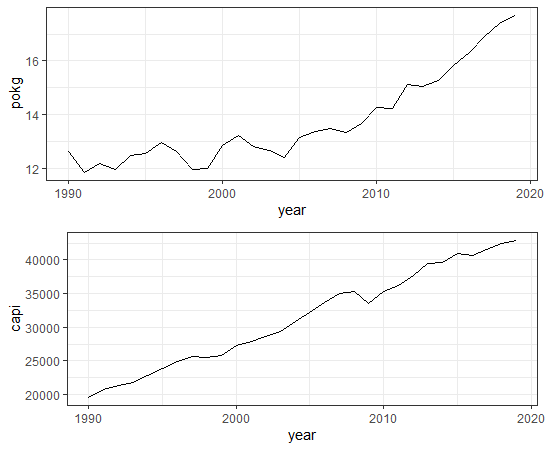The both, pokg: POULTRY KG_CAP and capi: per capita GDP have uptrend.

I will make 4 time-series regression models.

1. Static Model

2. Finite Distributed Lag Model

3. Static Model with Trend

4. Finite Distrubuted Lag Model with Trend.

Before make regression models, I load dynlm package.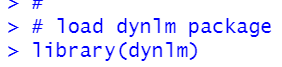All right, let's make those models.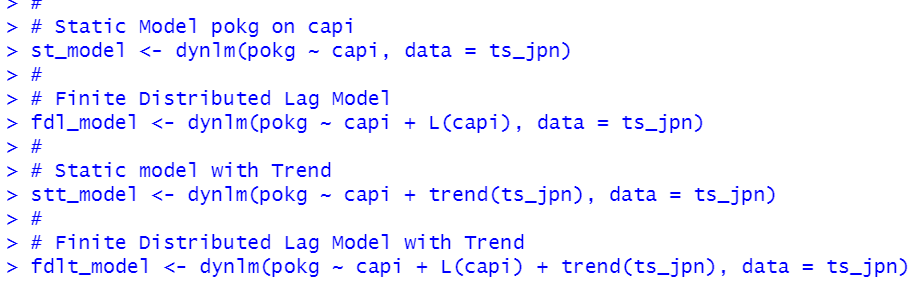I use stargazer package to make results table.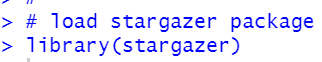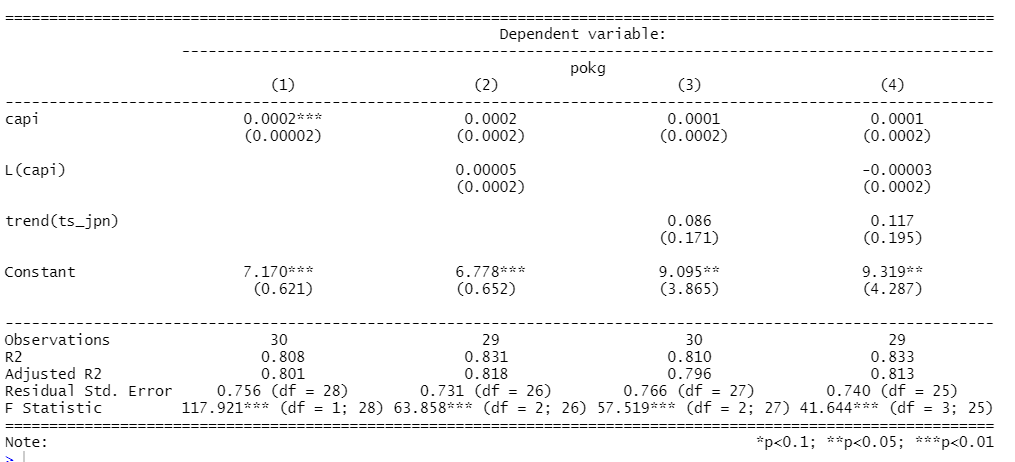We see capi has positive coefficients.

That's it. Thank you!

Next post is